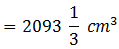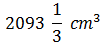Newbie

# The surface area of a solid sphere is 1256 cm square. It is cut into two hemispheres. Find the total surface area and the volume of a hemisphere. Take pie (π) = 3.14.

• 1

M.L Aggarwal book Important Question of class 10 chapter Based on Mensuration for ICSE BOARD.
The surface area of a solid sphere is given.
It is cut into two hemispheres. Find the total surface area and the volume of a hemisphere.
This is the Question Number 16, Exercise 17.3 of M.L Aggarwal.

Share

1.Given surface area of the sphere = 1256 cm2

4πr2 = 1256

4×3.14×r2 = 1256

r2 = 1256×/3.14×4

r2 = 100

r = 10 cm

Total surface area of the hemisphere = 3πr2

= 3×3.14×102

= 3×3.14×100

= 942 cm2

Hence the total surface area of the hemisphere is 942 cm2.

Volume of the hemisphere = (2/3)πr3

= (2/3)×3.14×103

= (2/3)×3.14×1000

= (2/3)×3140

= 6280/3Hence the volume of the hemisphere is• 5